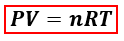# Solution: When 15.0 g of fluorite (CaF2) reacts with excess sulfuric acid, hydrogen fluoride gas is collected at 744 torr and 25.5°C. Solid calcium sulfate is the other product. What gas temperature is required to store the gas in an 8.63-L container at 875 torr?

###### Problem

When 15.0 g of fluorite (CaF2) reacts with excess sulfuric acid, hydrogen fluoride gas is collected at 744 torr and 25.5°C. Solid calcium sulfate is the other product. What gas temperature is required to store the gas in an 8.63-L container at 875 torr?

###### Solution

We are asked to calculate for the gas temperature is required to store the gas in an 8.63-L container at 875 torr. We can calculate the required temperature of the gas using the ideal gas equation:P = pressure, atm
V = volume, L
n = moles, mol
R = gas constant = 0.08206 (L·atm)/(mol·K)
T = temperature, K

In the ideal gas equation, the amount of the gas in moles is needed but the mass of CaF2 is given instead. We will have to calculate the moles of gas produced from the reaction first using the balanced reaction equation and mole to mole comparison.

Write the balanced reaction equation:View Complete Written Solution